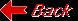Planet SaturnMass (kg) 5.688e+26 Mass (Earth = 1) 9.5181e+01 Equatorial radius (km) 60,268 Equatorial radius (Earth = 1) 9.4494e+00 Mean density (gm/cm^3) 0.69 Mean distance from the Sun (km) 1,429,400,000 Mean distance from the Sun (Earth = 1) 9.5388 Rotational period (hours) 10.233 Orbital period (years) 29.458 Mean orbital velocity (km/sec) 9.67 Orbital eccentricity 0.0560 Tilt of axis (degrees) 25.33 Orbital inclination (degrees) 2.488 Equatorial surface gravity (m/sec^2) 9.05 Equatorial escape velocity (km/sec) 35.49 Visual geometric albedo 0.47 Magnitude (Vo) 0.67 Mean cloud temperature -125C Atmospheric pressure (bars) 1.4 Atmospheric composition: Hydrogen 97%,Helium 3%